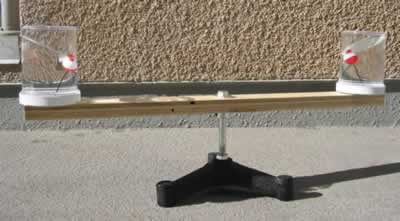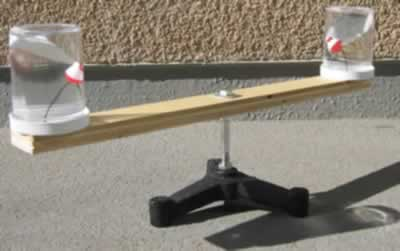## Visualizing Centripetal Acceleration

Author(s): Christopher Chui and Jim Mauch

Demonstration Equipment - Teacher's Guide
SED 695B; Fall 2005

1. Newton's laws predict the motion of most objects. As a basis for understanding this concept:
Students know applying a force to an object perpendicular to the direction of its motion causes the object to change direction but not speed (e.g., Earth's gravitational force causes a satellite in a circular orbit to change direction but not speed).
Students know circular motion requires the application of a constant force directed toward the center of the circle.
Students know how to solve problems in circular motion by using the formula for centripetal acceleration in the following form: a=v2/r.

Middle School

Unbalanced forces cause changes in velocity. As a basis for understanding this concept:
Students know a force has both direction and magnitude.
Students know that when the forces on an object are unbalanced, the object will change its velocity (that is, it will speed up, slow down, or change direction).

The velocity of an object is the rate of change of its position. As a basis for understanding this concept:
Students know the velocity of an object must be described by specifying both the direction and the speed of the object.
Students know changes in velocity may be due to changes in speed, direction, or both.Principles illustrated:

• Centripetal acceleration
• Centripetal force
• Inertia

Materials

'Circular Motion Paradox' demonstration kit. Flinn Scientific catalog AP6382

Water

Explanation of principles involved

• In order for there to be uniform circular motion, there must be a force acting at right angles to the instantaneous velocity of the object.
• This force is directed toward the center of the circle.
• This force (centripetal force) causes a change in direction , but not a change in speed.
• Velocity is a vector quantity, having both speed and direction. So a change in the direction is a change in velocity (acceleration).
• The resulting centripetal acceleration is in the same direction as the centripetal force
• The water in the cups 'piles up' on the outside edge due to inertia
• This results in a greater pressure near the outside edge compared to the inside edge
• The buoy shifts toward the lower pressure, pointing in the direction of the centripetal acceleration
 CIRCULAR MOTION PARADOX Radius of arm (cm) 22 Height of plastic jar (cm) 5.5 Radius of plastic jar (cm) 3.5 Diameter of plastic ball (cm) 2 Radius of arm (m) 0.22 Height of plastic jar (m) 0.055 Radius of plastic jar (m) 0.035 Radius of plastic ball (m) 0.01 Volume of water (m^3) 0.000211665 Mass of water (kg) 0.2116653 Assuming the spin is 1 revolution per second, this translates into 2(pi) radians per second. Then, velocity, v = r* omega (m/s) 1.382304 Acceleration = v^2/r (m/s^2) 8.685292493 Centripetal force = mv^2/r (Newtons) 1.838375041 Because there are two plastic jars having roughly the same mass, the centripetal force is doubled. Total centripetal force (Newtons) 3.676750082 The submerged plastic buoy is more difficult to analyze because we do not know the mass of the ball. The tension in the string can be estimated if we know the mass of the buoy. We will use mg + Tension = buoyant force, which is the mass of water displaced. The sideward swing of the buoy can be estimated to balance the centripetal force due to the buoy alone.

Procedure:

• Place water in the jars so that the buoys are suspended under the surface.
• Ask students to make predictions as to the movement of the buoys as the apparatus turns
• Spin the apparatus. Students can make observations of the inward movement of the buoys and discuss.
• Connections can be made to the effect of linear acceleration on a person in a car and centripetal acceleration as the car goes around a curve. Place a balloon in a car. Have the driver proceed to make a turn at a constant speed. The balloon will bend in the direction of centripetal acceleration.As the device turns, yo can see the water piling up on the outside edge of each cup. The buoy points in the direction of the centripetal acceleration.